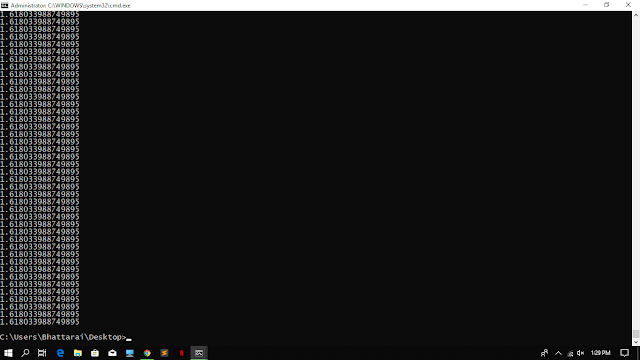### How to generate Golden Ratio by programming?

Golden ratio has been a fascinating topic for last more than 2400 years despite it does not have reliable evidence. Golden ratio is seen in the circle of Sunflower "flower". We can get golden ratio when we divide a line into two parts, the ratio of dividing the large part with small part is equal to the whole length divided by the longer part.

For a programmer it will be more fascinating to know how to code the golden ratio. The most easy way to do this is by using Fibonacci Series. We can use python code like this.

s = 0
a = 1
b = 1
for x in range(9999):
c = a + b
a = b
print (c/b)  #This is the golden ratio
b = cGolden ratio occurs in nature in different forms. Some of the examples are:
1. Flower Petals
3. Pine Cones
4. Shells
5. Spiral galaxies
6. Hurricanes
7. Fingers
8. Animal Bodies
9. DNA molecules

Thank you!
A blog by Saroj Bhattarai

1.2.https://knowyourmeme.com/photos/1076057-the-golden-ratio